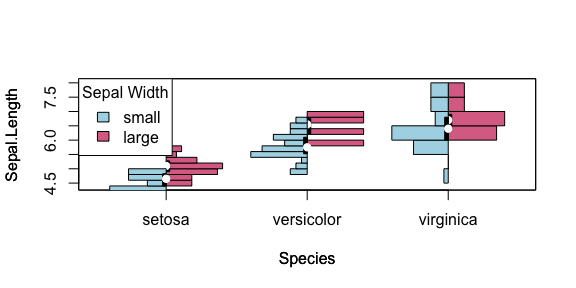# Customising Violin Plots with Histograms

#### 2022-12-09

While boxplots have become the de facto standard for plotting the distribution of data this is a vast oversimplification and may not show everything needed to evaluate the variation of data. This is particularly important for datasets which do not form a Gaussian “Normal” distribution that most researchers have become accustomed to.

While density plots are helpful in this regard, they can be less aesthetically pleasing than boxplots and harder to interpret for those familiar with boxplots. Often the only ways to compare multiple data types with density use slices of the data with faceting the plotting panes or overlaying density curves with colours and a legend. This approach is jarring for new users and leads to cluttered plots difficult to present to a wider audience.

Therefore violin plots are a powerful tool to assist researchers to visualise data, particularly in the quality checking and exploratory parts of an analysis. Violin plots have many benefits:

• Greater flexibility for plotting variation than boxplots
• More familiarity to boxplot users than density plots
• Easier to directly compare data types than existing plots

As shown below for the iris dataset, histogram plots show distribution information that the boxplot is unable to.

library("vioplot")
## Loading required package: sm
## Package 'sm', version 2.2-5.7: type help(sm) for summary information
## Loading required package: zoo
##
## Attaching package: 'zoo'
## The following objects are masked from 'package:base':
##
##     as.Date, as.Date.numeric
data(iris)
boxplot(iris$Sepal.Length[iris$Species=="setosa"], iris$Sepal.Length[iris$Species=="versicolor"], iris$Sepal.Length[iris$Species=="virginica"], names=c("setosa", "versicolor", "virginica"))
library("vioplot")
vioplot(iris$Sepal.Length[iris$Species=="setosa"], iris$Sepal.Length[iris$Species=="versicolor"], iris$Sepal.Length[iris$Species=="virginica"], names=c("setosa", "versicolor", "virginica"))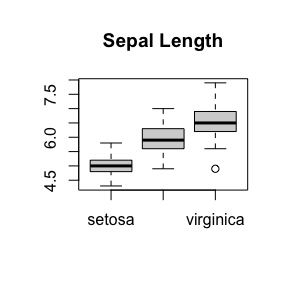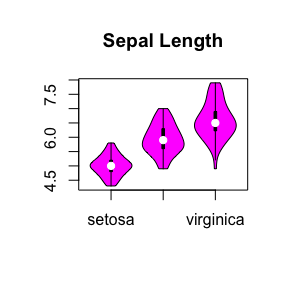## Plot Defaults

However as we can see here the plot defaults are not aesthetically pleasing, with a rather glaring colour scheme unsuitable for professional or academic usage. Thus the plot default colours have been changed as shown here:

vioplot(iris$Sepal.Length[iris$Species=="setosa"], iris$Sepal.Length[iris$Species=="versicolor"], iris$Sepal.Length[iris$Species=="virginica"], names=c("setosa", "versicolor", "virginica"), main = "Sepal Length")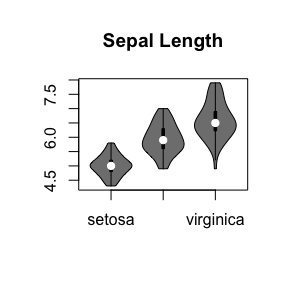# Histogram plot

Here we introduce a variant of the violin plot, using a mirrored bihistogram to show the distribution:

histoplot(iris$Sepal.Length[iris$Species=="setosa"], iris$Sepal.Length[iris$Species=="versicolor"], iris$Sepal.Length[iris$Species=="virginica"], names=c("setosa", "versicolor", "virginica"), main = "Sepal Length")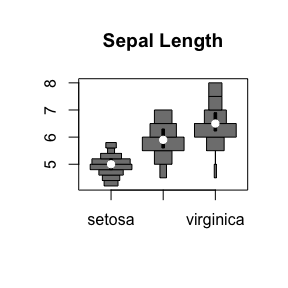## Plot colours: Histogram Fill

Plot colours can be further customised as with the original viooplot package using the col argument:

histoplot(iris$Sepal.Length[iris$Species=="setosa"], iris$Sepal.Length[iris$Species=="versicolor"], iris$Sepal.Length[iris$Species=="virginica"], names=c("setosa", "versicolor", "virginica"), main = "Sepal Length", col="lightblue")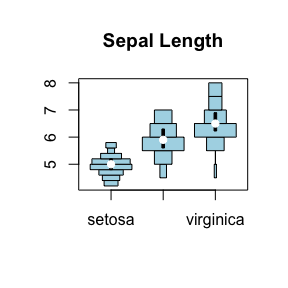### Vectorisation

The vioplot (0.2) function is unable to colour each histogram separately, thus this is enabled with a vectorised col in viooplot (0.3) and histoplot (0.4):

vioplot(iris$Sepal.Length[iris$Species=="setosa"], iris$Sepal.Length[iris$Species=="versicolor"], iris$Sepal.Length[iris$Species=="virginica"], names=c("setosa", "versicolor", "virginica"), main = "Sepal Length", col=c("lightgreen", "lightblue", "palevioletred"))
legend("topleft", legend=c("setosa", "versicolor", "virginica"), fill=c("lightgreen", "lightblue", "palevioletred"), cex = 0.5)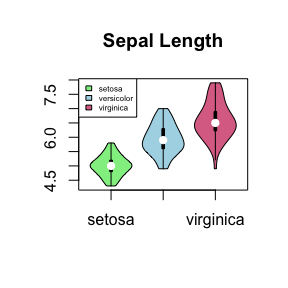histoplot(iris$Sepal.Length[iris$Species=="setosa"], iris$Sepal.Length[iris$Species=="versicolor"], iris$Sepal.Length[iris$Species=="virginica"], names=c("setosa", "versicolor", "virginica"), main = "Sepal Length", col=c("lightgreen", "lightblue", "palevioletred"))
legend("topleft", legend=c("setosa", "versicolor", "virginica"), fill=c("lightgreen", "lightblue", "palevioletred"), cex = 0.5)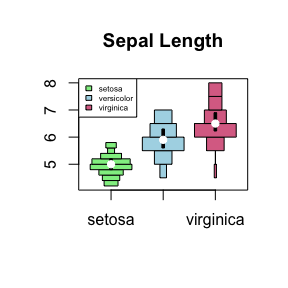## Plot colours: Violin Lines and Boxplot

Colours can also be customised for the histogram fill and border separately using the col and border arguments:

histoplot(iris$Sepal.Length[iris$Species=="setosa"], iris$Sepal.Length[iris$Species=="versicolor"], iris$Sepal.Length[iris$Species=="virginica"], names=c("setosa", "versicolor", "virginica"), main = "Sepal Length", col="lightblue", border="royalblue")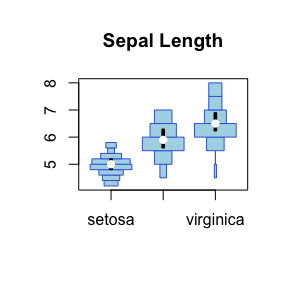Similarly, the arguments lineCol and rectCol specify the colors of the boxplot outline and rectangle fill. For simplicity the box and whiskers of the boxplot will always have the same colour.

histoplot(iris$Sepal.Length[iris$Species=="setosa"], iris$Sepal.Length[iris$Species=="versicolor"], iris$Sepal.Length[iris$Species=="virginica"], names=c("setosa", "versicolor", "virginica"), main = "Sepal Length", rectCol="palevioletred", lineCol="violetred")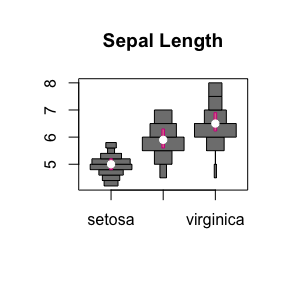The same applies to the colour of the median point with colMed:

histoplot(iris$Sepal.Length[iris$Species=="setosa"], iris$Sepal.Length[iris$Species=="versicolor"], iris$Sepal.Length[iris$Species=="virginica"], names=c("setosa", "versicolor", "virginica"), main = "Sepal Length", colMed="violet")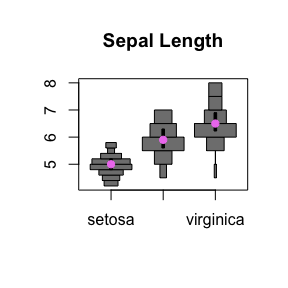### Combined customisation

These can be customised colours can be combined:

histoplot(iris$Sepal.Length[iris$Species=="setosa"], iris$Sepal.Length[iris$Species=="versicolor"], iris$Sepal.Length[iris$Species=="virginica"], names=c("setosa", "versicolor", "virginica"), main = "Sepal Length", col="lightblue", border="royalblue", rectCol="palevioletred", lineCol="violetred", colMed="violet")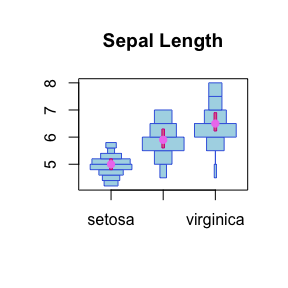### Vectorisation

These color and shape settings can also be customised separately for each histogram:

histoplot(iris$Sepal.Length[iris$Species=="setosa"], iris$Sepal.Length[iris$Species=="versicolor"], iris$Sepal.Length[iris$Species=="virginica"], names=c("setosa", "versicolor", "virginica"), main="Sepal Length (Equal Area)", areaEqual = T, col=c("lightgreen", "lightblue", "palevioletred"), border=c("darkolivegreen4", "royalblue4", "violetred4"), rectCol=c("forestgreen", "blue", "palevioletred3"), lineCol=c("darkolivegreen", "royalblue", "violetred4"), colMed=c("green", "cyan", "magenta"), pchMed=c(15, 17, 19))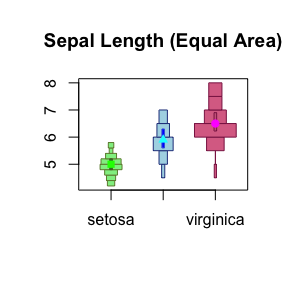## Split Bihistogram Plots

We set up the data with two categories (Sepal Width) as follows:

data(iris)
summary(iris$Sepal.Width) ## Min. 1st Qu. Median Mean 3rd Qu. Max. ## 2.000 2.800 3.000 3.057 3.300 4.400 table(iris$Sepal.Width > mean(iris$Sepal.Width)) ## ## FALSE TRUE ## 83 67 iris_large <- iris[iris$Sepal.Width > mean(iris$Sepal.Width), ] iris_small <- iris[iris$Sepal.Width <= mean(iris\$Sepal.Width), ]

A direct comparision of 2 datasets can be made with the side argument and add = TRUE on the second plot:

histoplot(Sepal.Length~Species, data=iris_large, col = "palevioletred", plotCentre = "line", side = "right")
histoplot(Sepal.Length~Species, data=iris_small, col = "lightblue", plotCentre = "line", side = "left", add = T)
title(xlab = "Species", ylab = "Sepal Length")
legend("topleft", fill = c("lightblue", "palevioletred"), legend = c("small", "large"), title = "Sepal Width")